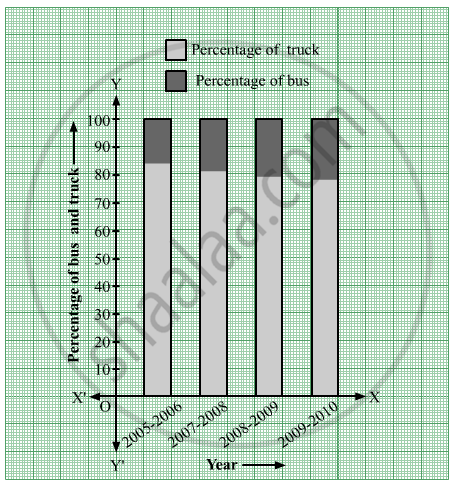Advertisement Remove all ads

# The Following Table Shows the Number of Buses and Trucks in Nearest Lakh Units. Draw Percentage Bar-diagram. (Approximate the Percentages to the Nearest Integer) - Algebra

Graph

The following table shows the number of Buses and Trucks in nearest lakh units. Draw percentage bar-diagram. (Approximate the percentages to the nearest integer)

 Year No  of trucks No of buses 2005-2006 2007-2008 2008-2009 2009-2010 47      56       60      63 9         13         16         18
Advertisement Remove all ads

#### Solution

The following table shows the number of Buses and Trucks in nearest lakh units.

 Year No  of trucks Percentage of trucks No of buses Percentage of buses 2005-2006 47 =47(47+9)×100=470056=83.92≈84 9 =9(47+9)×100=90056=16.07≈16 2007-2008 56 =56(56+13)×100=560069=81.15≈81 13 =13(56+13)×100=130069=18.84≈19 2008-2009 60 =60(60+16)×100=600076=78.94≈79 16 =16(60+16)×100=160076=21.05≈21 2009-2010 63 =63(63+18)×100=630081=77.77≈78 18 =18(63+18)×100=180081=22.22≈22

The percentage bar-diagram of the above table is as follows:Is there an error in this question or solution?
Advertisement Remove all ads
Advertisement Remove all ads
Share
Notifications

View all notifications

Forgot password?